Introduction: Blood Relations

# Introduction: Blood Relations - Notes | Study General Aptitude for GATE - Mechanical Engineering

 Table of contentsIntroductionBlood Relations: Type of QuestionsBlood Relations: Tips & Strategies1 Crore+ students have signed up on EduRev. Have you?

Introduction

• The questions related to blood relation involve various family members and their relations.
• The questions provide some information related to the different family members and the candidates are required to identify the relationship between those particular members.
• The blood relations questions might be of different forms which are explained in detail below.

Blood Relations: Type of Questions

• The blood relation questions have several variations to test the understanding and interpretation capabilities of the candidates.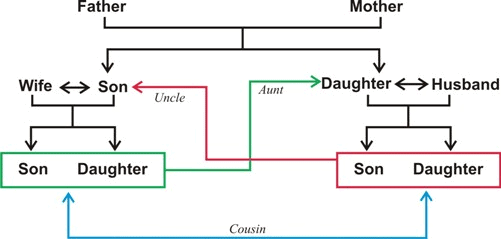The question types are divided into three categories as:
• Direct conversation-based questions
• Puzzle based questions
• Symbols (or Code) based questions
• Though the questions have these aforementioned types, solving the problems and the ultimate aim of the questions always remain unchanged.
• Whatever the questions are, the candidates are always required to identify the relationship between two particular entities.

The types can further be classified as:

• Single person blood relation: It involves the relationship between two people only. The questions can be either direct or indirect.
• Mixed blood relation: The relation between several members and hierarchy are considered. The different variations of questions are explained below along with an example each.

Direct Conversation Based Questions

• In direct conversation-based blood relation questions, a problem statement (in the form of conversation) is given and a particular relation is asked from the conversation.
• In general, these questions are single person blood relation types, i.e. involving the relationship between two persons only.
• An example will illustrate this better.

Example: Showing a photo of a man, Pinki says,” His mother’s only daughter is my mother”. How is Pinki related to the man in the photo?
Solution:
Analysing the sentence, it can be deduced that Pinki’s mother is the sister of the man in the photo. So, the man is the Uncle of Pinki or Pinki is the niece of that man.

Question for Introduction: Blood Relations
Try yourself:Pointing to a photograph of a boy Suresh said, "He is the son of the only son of my mother." How is Suresh related to that boy?

Puzzle Based Questions

• In this type of questions, the statements are given in the form of puzzles.
• The relations are mostly of mixed blood relation type and the candidates are required to decode the entire statement and identify the relationship between the given entities.
• Here is an illustration to explain this well.

Example: In a family of W, X, Y and Z, W and X are brothers; while Y and Z are sisters. How are X and Y related if W’s son is Z’s brother?
Solution:
From the statement, a simple diagram can be sketched to analyse the relation between Y and Z.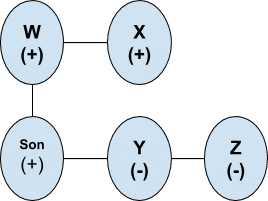• As the total generations in the question are two, the number of rows in the diagram will also be two.
• The sign (+) implies male, while (-) implies female.
• From the diagram it is clear that Z’s and Y’s father is W. As X is W’s brother, it can be said that X is Y’s uncle.

Question for Introduction: Blood Relations
Try yourself:If A is the brother of B; B is the sister of C; and C is the father of D, how D is related to A?

Symbol Based Questions

• Also known as coded blood relation questions, these involve a set of codes. The candidates are required to decode the sentences (or codes) to identify the relation of the given entities.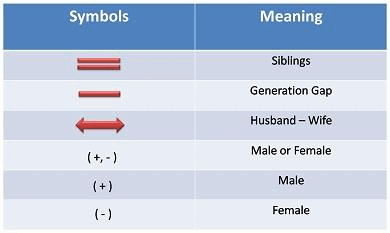Example: Consider the following statements and answer the given question.

• If L + M => L is the husband of M
• L / M => L is the sister of M
• L + M => L is the son of Y

How is W and Z related from the following statement: W + X * Y / Z?
Solution: This can be solved by dividing the question and solving from left to right. Now a simple diagram can be made by mentioning the gender and their relations as given below: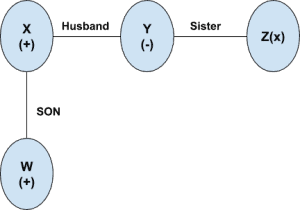Thus, it is easily seen that W is the nephew of Z.

These were the most important types of blood relation question that are frequently asked in the CAT exam. Solving different variation of the related question is very crucial to be able to increase the confidence, speed and accuracy while solving the actual CAT paper.

Question for Introduction: Blood Relations
Try yourself:Pointing towards a man, a woman said, "His mother is the only daughter of my mother." How is the woman related to the man?

Blood Relations: Tips & Strategies

• As the topic is repeated every year in the CAT exam, the candidates are suggested to be thorough with the basics and other related concepts to increase their chances of scoring more in the exam.
• Here some important tips and strategies are given to help the CAT aspirants ace the blood relation question with ease.

Be Thorough with the Blood Relation Chart

• It is suggested to get well versed with the blood relation chart to be able to relate and answer the questions easily.
• Here is a chart that details the entire family relation.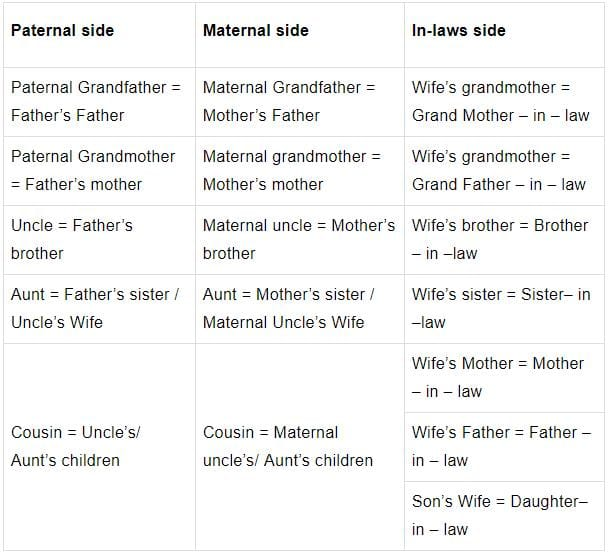Be Confident with the Family Tree

• The questions require the candidates to be thorough with the basic relations within a family. In a family tree, the people belonging to the same generation are placed in the same row, while people from different generations are placed in successive rows. The males can be marked as “+” and the females as “-”.
• Drawing a proper family tree for every question is extremely crucial as it will help to identify and analyse the statement easily. The family tree is used above for solving the example questions and it can be seen that the problem becomes much simpler after the tree is drawn accurately.
• However, if the family tree is incorrectly drawn, one might identify a wrong relationship and eventually lose score.

Break the Question Statements

• Numerous candidates get confused and fail to analyse the complete question statement at once. This is mainly because the blood relation question statements are sometimes long and confusing.

Example: That girl is Ramu’s sister’s uncle’s wife’s daughter. How is that girl related to Ramu?
Solution:
This question seems confusing initially; but, breaking the sentence into parts and then analysing the relation can be extremely easy. Now this sentence can be broken as:

• Ramu’s sister’s uncle = Ramu’s uncle
• Ramu’s uncle’s wife’s son = Ramu’s cousin
• Therefore, that girl is the cousin of Ramu

Relate the Problems Personally

• The blood relation concepts can be solved quickly and easily if the questions are related personally.
• So, relate the problems to the self and start analysing the questions strategically.
• Here is an example to understand the importance of relating the problems to self:

Example: That man is Raju’s sister’s mother’s brother. How is that boy related to Raju?
Solution:
This question can be either solved with a family tree or by breaking the sentence and relating the relations personally. So, if this question is solved by personal relation, the sentences become easy to analyse.

• Assume that you are Raju himself.
• Therefore, that man is your (Raju’s) maternal uncle

It should be noted that this approach should only be taken when the question statement is short. For questions with a long sentence, it is suggested to draw the family tree to easily solve the questions.

Never Assume Gender

• It is always suggested to avoid assuming the gender of a person based on the given name in the question statement.
• This sometimes leads to losing some crucial marks as the questions are set to test the overall cognitive skills of the candidates.
Example: Tina is the sister of Sukhwinder and Sukhwinder is the brother of Roshan. Then how is Roshan related to Tina?
Solution:
• From the above statement, the gender of Roshan is unclear and creates confusion about the same.
• Some might consider the name Roshan as male while others might consider it as female.
• So, coming to a conclusion from the given statement is impossible and that the question requires more information.

Question for Introduction: Blood Relations
Try yourself:P is the mother of K; K is the sister of D; D is the father of J. How is P related to J?
Question for Introduction: Blood Relations
Try yourself:If A \$ B means A is the brother of B; A @ B means A is the wife of B; A # B means A is the daughter of B and A * B means A is the father of B, which of the following indicates that U is the father-in-law of P?

The document Introduction: Blood Relations - Notes | Study General Aptitude for GATE - Mechanical Engineering is a part of the Mechanical Engineering Course General Aptitude for GATE.
All you need of Mechanical Engineering at this link: Mechanical Engineering

## General Aptitude for GATE

73 videos|86 docs|108 tests
 Use Code STAYHOME200 and get INR 200 additional OFF

## General Aptitude for GATE

73 videos|86 docs|108 tests

Track your progress, build streaks, highlight & save important lessons and more!

,

,

,

,

,

,

,

,

,

,

,

,

,

,

,

,

,

,

,

,

,

;